# Numerically evaluating a theta function

Theta functions pop up throughout pure and applied mathematics. For example, they’re common in analytic number theory, and they’re solutions to the heat equation.

Theta functions are analogous in some ways to trigonometric functions, and like trigonometric functions they satisfy a lot of identities. This post will comment briefly on an identity that makes a particular theta function, θ3, easy to compute numerically. And because there are identities relating the various theta functions, a method for computing θ3 can be used to compute other theta functions.

The function θ3 is defined bywhereHere’s a plot of θ3 with t = 1 + i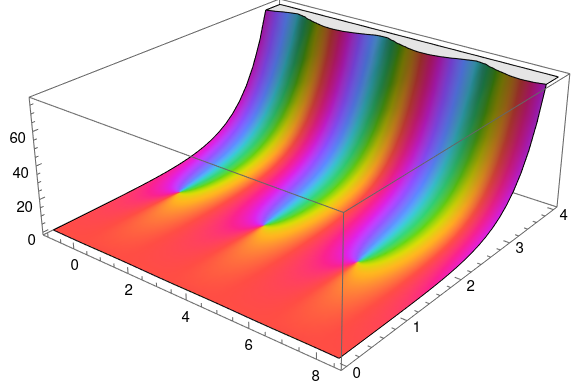produced by the following Mathematica code:


q = Exp[Pi I (1 + I)]
ComplexPlot3D[EllipticTheta[3, z, q], {z, -1, 8.5 + 4 I}]


An important theorem tells usThis theorem has a lot of interesting consequences, but for our purposes note that the identity has the formThe important thing for numerical purposes is that t is on one side and 1/t is on the other.

Suppose t = a + bi with a > 0 and b > 0. ThenNow if b is small, |q| is near 1, and the series defining θ3 converges slowly. But if b is large, |q| is very small, and the series converges rapidly.

So if b is large, use the left side of the theorem above to compute the right. But if b is small, use the right side to compute the left.

# Architecture and Math

I recently received a review copy of Andrew Witt’s new book Formulations: Architecture, Mathematics, and Culture.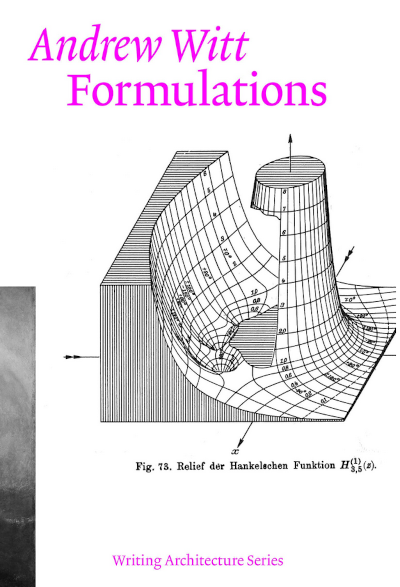The Hankel function on the cover is the first clue that this book contains some advanced mathematics. Or rather, it references some advanced mathematics. I’ve only skimmed the book so far, but I didn’t see any equations.

Hankel functions are defined in terms of Bessel functions:where J is the Bessel function of the first kind, Y is the Bessel function of the second kind, and ν is the order. I’ve only ever seen one subscript on Hankel functions, but my suspicion is that the two subscripts allow for different orders on J and Y. That is, my guess is thatHere’s my quick attempt to reproduce the cover art in Mathematica:

    H[m_, n_, z_] := BesselJ[m, z] + I BesselY[n, z]
ComplexPlot3D[H[3, 5, z], {z, -4 - 4 I, 5 + 5 I}]


This produces the following plot.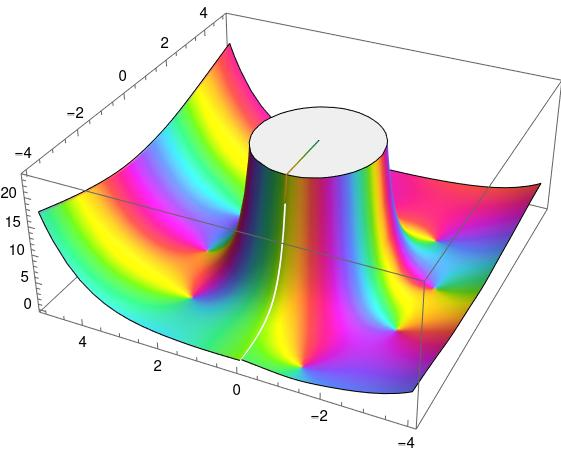I’m amazed that people ever had the patience and skill to manually make plots like the one on the cover of Witt’s book.

While thumbing through the book I noticed some curves that I recognized as Lissajous curves. Turns out these were curves made by Lissajous himself. The plots were accompanied by the mechanical devices Lissajous used to draw them.

# The exception case is normal

Sine and cosine have power series with simple coefficients, but tangent does not. Students are shocked when they see the power series for tangent because there is no simple expression for the power series coefficients unless you introduce Bernoulli numbers, and there’s no simple expression for Bernoulli numbers.

The perception is that sine and cosine are the usual case, and that tangent is exceptional. That’s because in homework problems and examples, sine and cosine are the usual case, and tangent is exceptional. But as is often the case, the pedagogically convenient case is the exception rather than the rule.

Of the six common trig functions—sine, cosine, tangent, secant, cosecant, cotangent—sine and cosine are the only ones with power series not involving Bernoulli numbers. The power series for most of the common trig functions require Bernoulli numbers.

## Details

The functions cosecant and cotangent have a singularity at 0, so the power series for these functions at 0 are Laurant series rather than Taylor series, i.e. they involve negative as well as positive exponents. We will look at z csc(z) and z cot(z) because multiplying by z removes the singularity.The series for secant doesn’t use Bernoulli numbers directly but rather Euler numbers. However, Bernoulli and Euler numbers are closely related.# The zeta distribution

The previous post on the Yule-Simon distribution mentioned the zeta distribution at the end. This is a powerlaw distribution on the positive integers with normalizing constant given by the Riemann zeta distribution. That is, the zeta distribution has density

f(k; s) = ks / ζ(s).

where k is a positive integer and s > 1 is a fixed parameter.

For s > 1, the zeta function is defined as the sum of the positive integers to the power negative s, so ζ(s) is essentially defined as the normalizing constant of the zeta distribution.

I wanted to make a couple comments about this. First, it shows that the zeta function appears in applications outside of number theory. Second, when working with the zeta distribution, it would be useful to have an estimate for ζ(s).

## Zeta function bounds

The integral test in calculus is typically presented as a way to test whether an infinite sum converges. This is a shame. In analysis as in statistics, estimation is better than testing. Testing throws away continuous information and replaces it with a single bit.

Let f(x) be a decreasing function; we have in mind f(x) = 1/xs. Then for any n,To estimate a sum over k, we sum the first n terms directly and apply the inequalities above for the rest. Typically n will be small, maybe 0 or 1. A larger value of n will give more accurate bounds but at the expense of a more complicated expression.

When f(x) = 1/xs and n is at least 1, we haveThis saysThis gives a good lower bound but a bad upper bound when we choose n = 1.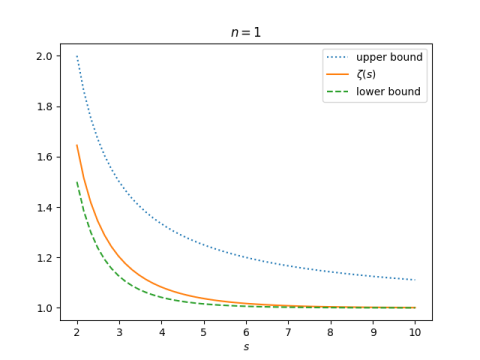But it gives a much better upper bound when we choose n = 2.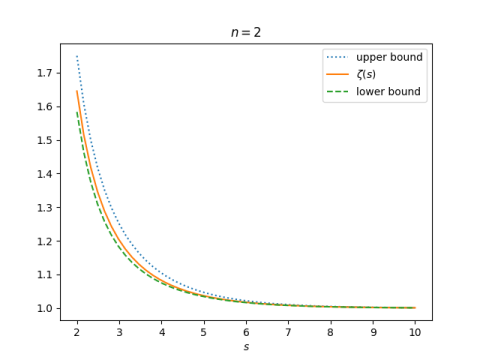# 3D Go with identities

Let’s play a game that’s something like Go in three dimensions. Our game is played on the lattice of points in 3D that have integer coordinates. Someone places stones on two lattice points, and your job is to create a path connecting the two stones by adding stones to neighboring locations.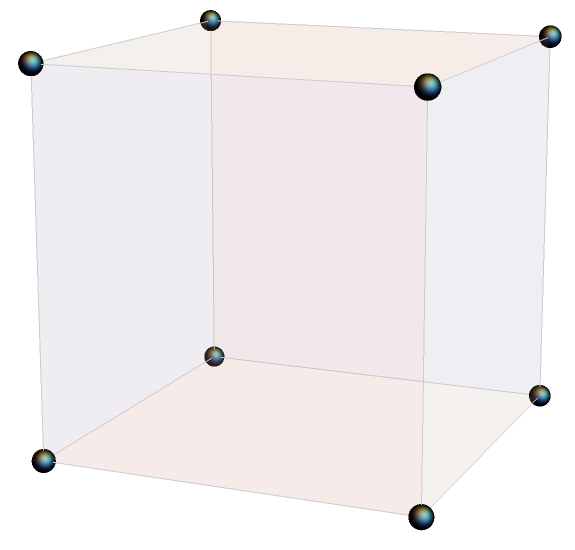## Game cube

We don’t really have a game “board” since we’re working in three dimensions. We have a game scaffold or game cube. And we can’t just put our stones down. We have to imagine the stones has having hooks that we can use to hang them where pieces of scaffolding come together.

## Basic moves

Now for the rules. Our game is based on identities for a function with depending on three parameters: a, b, and c. A location in our game cube is a point with integer coordinates (i, j, k) corresponding to function parameters

(a+i, b+j, c+k).

If there is a stone at (i, j, k) then we are allowed to place stones at neighboring locations if there is a function identity corresponding to these points. The function identities are the “contiguous relations” for hypergeometric functions. You don’t need to know anything about hypergeometric functions to play this game. We’re going to leave out a lot of detail.

Our rule book for identities will be Handbook of Mathematical Function by Abramowitz and Stegun, widely known as A&S. You can find a copy online here.

For example, equation 15.2.10 from A&S relates

F(a, b; c; z)

to

F(a-1, b; c; z)

and

F(a+1, b; c; z).

So if we have a stone at (i, j, k) we can add stones at (i-1, j, k) and (i+1, j, k). Or we could add stones at (i+1, j, k) and (i+2, j, k). You can turn one stone into a row of three stones along the first axis overlapping the original stone.

Equation 15.2.11 lets you do something analogous for incrementing the 2nd coordinate, and Equation 15.2.12 lets you increment the 3rd coordinate. So you could replace a stone with a row of three stones along the second or third axes as well.

This shows that it is always possible to create a path between two lattice points. If you can hop between stones a distance 1 apart, you can create a path that will let you hop between any two points.

There are other possible steps. For example, equation 15.2.25 has the form

F(a, b; c; z) + … F(a, b-1; c; z)  + … F(a, b; c+1; z) = 0

I’ve left out the coefficients above, denoting them by ellipses. (More on that below.) This identity says you don’t just have to place stones parallel to an axis. For example, you can add a stone one step south and another one step vertically in the same move.

The previous section showed that it’s always possible to create a path between two lattice points. This section implies the finding the shortest path between two points might be a little more interesting.

## Applications

There are practical applications to the game described in this post. One reason hypergeometric functions are so useful is that they satisfy a huge number of identities. There are libraries of hypergeometric identities, and there are still new identities to discover and new patterns to discover for organizing the identities.

The moves in the game above can be used to speed up software. Hypergeometric functions are expensive to evaluate, and so it saves time to compute a new function in terms of previously computed functions rather than having to compute it from scratch.

I used this trick to speed up clinical trial simulations. Consecutive steps in the simulation required evaluating contiguous hypergeometric functions, so I could compute one or two expensive functions at the beginning of the simulation and bootstrap them to get the rest of the values I needed.

## Coefficients

I’d like to close by saying something about the coefficients in these identities. The coefficients are complicated and don’t have obvious patterns, and so they distract from the underlying structure discussed here. You could stare at a list of identities for a while before realizing that apart from the coefficients the underlying relationships are simple.

All the coefficients in the identities cited above are polynomials in the variables a, b, c, and z. All have degree at most 2, and so they can be computed quickly.

The identities discussed here are often called linear relations. The relations are linear in the hypergeometric functions, but the coefficients are not linear.

# How to put a series in hypergeometric form

I skipped a step in the previous post, not showing how a series was put into the form of a hypergeometric function.

Actually I skipped two steps. I first said that a series was not obviously hypergeometric, and yet at first glance it sorta is.

I’d like to make up for both of these omissions, but with a simpler example. It looks like a hypergeometric series, and it is, but it’s not the one you might think. The example will be the function below.## Rising powers

Hypergeometric functions are defined by series whose coefficients are rising powers.

The nth rising power of a is

(a)n = a (a+1) (a + 2) … (a + n – 1).

More on rising powers here. The coefficient of xn in a series defining a hypergeometric function is a ratio of nth rising powers of constants.

## Why the example isn’t obvious

You can write factorials as rising powers: n! = (1)n. The binomial coefficient in our example is a ratio of rising powers:

(5n)! = (1)5n

in the numerator and

n! (4n)! = (1)n (1)4n

in the denominator. So why aren’t we done? Because although these are all rising powers, they’re not all nth rising powers. The subscripts on the (a)n are all different: 5n, 4n, and n.

So is our function not hypergeometric? It is, but we’ll have to introduce more parameters.

## Rational polynomials

Another way to define hypergeometric series is to say that the ratio of consecutive coefficients is a rational function of the index n. This is very succinct, but not as explicit as the definition in terms of rising powers, though they’re equivalent.

In addition to brevity, this definition has another advantage: the hypergeometric parameters are the negatives of the zeros and poles of said rational function. The zeros are the upper parameters and the poles are the lower parameters.

This is how you put a series in hypergeometric form, if it has such a form. It’s also how you test whether a series is hypergeometric: a series is hypergeometric if and only if the ratio of consecutive terms is a single rational function of the index.

## Back to our example

The ratio of the (n+1)st term to the nth term in our series isand the final expression is a rational function of n. We can read the hypergeometric function parameters directly from this rational function. The upper parameters are 1, 4/5, 3/5, 2/5, and 1/5. The lower parameters are 1, 3/4, 2/4, 1/4, and 1. Identical factors in the upper and lower parameters cancel each other out, so we can remove the 1 from the list of upper parameters and remove one of the 1’s from the list of lower parameters .

So our example function isThe order of the upper parameters doesn’t matter, and neither does the order of the lower parameters, though it matters very much which ones are upstairs and which are downstairs. The subscript to the left of the F gives the number of upper parameters and the subscript to the right gives the number of lower parameters. These subscripts are redundant since you could just count the number of parameters between the semicolons, but they make it easier to tell at a glance what class of hypergeometric function you’re working with.

## Spot check

Let’s evaluate our original series and our hypergeometric series at a point to make sure they agree. I chose x = 0.01 to stay within the radius of convergence of the hypergeometric series.

Both

    Sum[Binomial[5 n, n] (0.01^n)/n!, {n, 0, Infinity}]

and

    HypergeometricPFQ[
{1/5, 2/5, 3/5, 4/5},
{1/4, 2/4, 3/4, 1},
0.01*3125/256]


return the same value, namely 1.05233.

## Related posts

 The extra factor of n! in the denominator of hypergeometric functions complicates things. It seems like an unfortunately historical artifact to me, but maybe it’s a net benefit for reasons I’m not thinking of. When we look at zeros of the numerator and denominator, we have a single 1 on top and three ones on bottom. The 1 on top cancels out one of the 1s on bottom, but why don’t we have two 1s in the lower parameters? Because one of them corresponds to the extra n! in the denominator.

# Quintic trinomial root

This post looks at an exercise from Special Functions, exercise 6 in Appendix E.

Suppose that xm+1 + axb = 0. Show thatUse this formula to find a solution to x5 + 4x + 2 = 0 to four decimal places of accuracy. When m = 0 this series reduces to the geometric series. Write this sum as a hypergeometric series.

• It’s a possibly useful result.
• It’s an application of Lagrange’s inversion formula; that’s where the series comes from.
• The equation has m+1 roots. What’s special about the root given by the series?
• The sum is not obviously hypergeometric.
• What happens when the sum diverges? Is it a useful asymptotic series? Does the analytic continuation work?

I want to address the last two points in this post. Maybe I’ll go back and address the others in the future. To simplify things a little, I will assume m = 4.

The parameters of the hypergeometric series are not apparent from the expression above, nor is it even apparent how many parameters you need. It turns out you need m upper parameters and m-1 lower parameters. Since we’re interested in m = 4, that means we have a hypergeometric function of the form 4F3.We can evaluate this expression in Mathematica as

    f[a_, b_] := (b/a) HypergeometricPFQ[
{1/5, 2/5, 3/5, 4/5},
{1/2, 3/4, 5/4},
-3125 b^4 / (256 a^5)
]


When we evaluate f[4, -2] we get -0.492739, which is the only real root of

x5 + 4x + 2 = 0.

Recall that the sign on b is negative, so we call our function with b = -2.

Now lets, try another example, solving for a root of

x5 + 3x – 4 = 0.

If we plug a = 3 and b = 4 into the series at the top of the post, the series diverges immediately, not giving a useful asymptotic series before it diverges.

The series defining our hypergeometric function diverges for |z| > 1, and we’re evaluating it at z = -3125/243. However, the function can be extended beyond the unit disk by analytic continuation, and when we ask Mathematica to numerically evaluate the root with by running

    N{ f[3, 4] ]

we get 1, which is clearly a root of x5 + 3x – 4.

# Numbering the branches of the Lambert W function

The previous post used the Lambert W function to solve an equation that came up in counting partitions of an integer. The first solution found didn’t make sense in context, but another solution, one on a different branch, did. The default branch k = 0 wasn’t what we were after, but the branch k = -1 was.

## Branches 0 and -1

The branch corresponding to k = 0 is the principal branch. In mathematical notation, the k is usually a subscript, i.e W0(z) is the principal branch of the Lambert W function. For real z ≥ -1/e it returns a real value. It’s often what you want, and that’s why it’s the default in software implementations such as SciPy and Mathematica. More on that below.

The only other branch that returns real values for real inputs is W-1 which returns real values for -1/ez < 0.

If you’re working strictly with real numbers, you only need to be concerned with branches 0 and -1. If branch 0 doesn’t give you what you expect, try branch -1, if your argument is negative.

## SciPy and Mathematica

SciPy implements Wk with lambertw(z). The parameter k defaults to 0.

The Mathematica function ProductLog[z] implements W0(z), and ProductLog[k, z] implements Wk.

Note that Mathematica and Python list their arguments in opposite orders.

## Branch numbering

The branches are numbered in order of their imaginary parts. That is, The imaginary part of Wk (z) is an increasing function of k. For example, here is a list of the numerical values of the imaginary parts of Wk (1) for k running from -3 to 3.

    Table[N[Im[ProductLog[k, 1]]], {k, -3, 3}]
{-17.1135, -10.7763, -4.37519, 0., 4.37519, 10.7763, 17.1135}


Note the zero in the middle because W0(1) is real.

## Recovering k

Given z and a value Wk (z) with k unknown, you can determine k withwith the exception that if the expression above is 0 and -1/ez < 0 then k = -1. See .

For example, let z = 1 + 2i and w = W3 (z).

    z = 1 + 2 I
w = ProductLog[3, z]


Now pretend you don’t know how w was computed. When you compute

    N[(w + Log[w] - Log[z])/(2 Pi I)]

the result is 3.

## Partition example

The discussion of the W function here grew out of a problem with partitions. Specifically, we wanted to solve for n such thatis approximately a trillion. We found in the previous post that solutions of the equationare given byOur partition problem corresponds to a = 1/√48, b = -1, c = π √(2/3), and d = 1/2. We found that the solution we were after came from the k = -1 branch.

    N[( (b/(c d)) ProductLog[-1, (x/a)^(d/b) c d /b])^(1/d)]
183.852


Even though our final value was real, the intermediate values were not. The invocation of W-1 in the middle returns a complex value:

    N[ProductLog[-1, ((x/a)^(d/b) c d /b)^(1/d)]]
-32.5568 - 3.24081 I


We weren’t careful about specifying ranges and branch cuts, but just sorta bulldozed our way to a solution. But it worked: it’s easy to verify that 183.852 is the solution we were after.

***

 Unwinding the branches of the Lambert W function by Jeffrey, Hare, and Corless. The Mathematical Scientist 21, 1&ndash;7 (1996)

# Solving equations with Lambert’s W function

In the previous post we wanted to find a value of n such that f(n) = 1012 whereand we took a rough guess n = 200. Turns out f(200) ≈ 4 × 1012 and that was good enough for our purposes. But what if we wanted to solve f(n) = x accurately?

We will work our way up to solving f(n) = 1012 exactly using the Lambert W function.

## Lambert’s W function

If you can solve one equation involving exponential functions you can bootstrap your solution solve a lot more equations.

The Lambert W function is defined to be the function W(x) that maps each x to a solution of the equation

w exp(w) = x.

This function is implemented Python under scipy.special.lambertw. Let’s see how we could use it solve similar equations to the one that define it.

## Basic bootstrapping

Given a constant c we can solve

cw exp(w) = x

simply by solving

w exp(w) = x/c,

which says w = W(x/c).

And we can solve

w exp(cw) = x

by setting y = cw and solving

y exp(y) = cx.

and so cw = W(cx) and w = W(cx)/c.

Combining the two approaches above shows that the solution to

aw exp(bw) = x

is

w = W(bx/a) / b.

We can solve

exp(w) / w = x

by taking the reciprocal of both sides and applying the result above with a = 1 and b = -1.

More generally, we can solve

wc exp(w) = x

by raising both sides to the power 1/c; the reciprocal the special case c = 1.

Similarly, we can solve

w exp(wc) = x

by setting y = wc , changing the problem to

y-1/c exp(y) = x.

## Putting it all together

We’ve now found how to deal with constants and exponents on w, inside and outside the exponential function. We now have all the elements to solve our original problem.

We can solve the general equationwithThe equation at the top of the post corresponds to a = 1/√48, b = -1, c = π √(2/3), and d = 1/2.

We can code this up in Python as follows.

    from scipy.special import lambertw

def soln(a, b, c, d, x):
t = (x/a)**(d/b) *c*d/b
return (lambertw(t)*b/(c*d))**(1/d)


We can verify that our solution really is a solution by running it though

    from numpy import exp, pi

def f(a, b, c, d, w):
return a*w**b * exp(c*w**d)


to make sure we get our x back.

When we run

    a = 0.25*3**-0.5
b = -1
c = pi*(2/3)**0.5
d = 0.5
x = 10**12

w = soln(a, b, c, d, x)
print(f(a, b, c, d, w))


we do indeed get x back.

    a = 0.25*3**-0.5
b = -1
c = pi*(2/3)**0.5
d = 0.5

w = soln(a, b, c, d, 10)
print(f(a, b, c, d, w))


When we take a look at the solution w, we get 1.443377079584187e-13. In other words, we get a number near zero. But our initial guess was w = 200. What went wrong?

Nothing went wrong. We got a solution to our equation. It just wasn’t the solution we expected.

The Lambert W function has multiple branches when viewed as a function on complex numbers. It turns out the solution we were expecting is on the branch SciPy denotes with k = -1. If we add this to the call to lambertw above we get the solution 183.85249773880142 which is more in line with what we expected.

# Mathematical plot twist: q-binomial coefficients

The simplest instance of a q-analog in math is to replace a positive integer n by a polynomial in q that converges to n as q → 1. Specifically, we associate with n the polynomial

[n]q = 1 + q + q² + q³ + … + qn-1.

which obviously converges to n when q → 1.

More generally we can associate with a real number a the rational polynomial

[a]q = (1 – qa) / (1 – q).

When a is a positive integer, the two definitions are the same. When a is not an integer, you can see by L’Hôpital’s rule that

limq → 1 [a]q = a.

## Motivation

Why would you do this? I believe the motivation was to be able to define a limit of a series where the most direct approach fails or is difficult to work with. Move over to the land of q-analogs, do all your manipulations there where requiring |q| < 1 avoids problems with convergence, then take the limit as q goes to 1 when you’re done.

## q-factorials and q-binomial coefficients

The factorial of a positive integer is

n! = 1 × 2 × 3 × … × n

The q-factorial of n replaces each term in the definition of factorial with its q-analog:

[n]q! = q! q! q! … [n]q!

(It helps to think of []q! as a single operator defining the q-factorial as above. This is not the factorial of [n]q.

n! / (k! (nk)!)

and define the q-binomial coefficient by replacing factorials with q-factorials.

[n]q! / ([k]q! [nk]q!)

You can apply the same process to rising and falling powers, and then replace the rising powers in the definition of a hypergeometric function to define q-analogs of these functions.

(The q-analogs of hypergeometric functions are confusingly called “basic hypergeometric functions.” They are not basic in the sense of simple examples; they’re not examples at all but new functions. And they’re not basic in the sense of a basis in linear algebra. Instead, the name comes from q being the base of an exponent.)

## Plot twist

And now for the plot twist promised in the title.

I said above that the q-analogs were motivated by subtle problems with convergence. There’s the idea in the background that eventually someone is going to let q approach 1 in a limit. But what if they don’t?

The theorems proved about q-analogs were first developed with the implicit assumption that |q| < 1, but at some point someone realized that the theorems don’t actually require this, and are generally true for any q. (Maybe you have to avoid q = 1 to not divide by 0 or something like that.)

Someone observed that q-analog theorems are useful when q is a prime power. I wonder who first thought of this and why. It could have been inspired by the notation, since q often denotes a prime power in the context of number theory. It could have been as simple as a sort of mathematical pun. What if I take the symbol q in this context and interpret it the way q is interpreted in another context?

Now suppose we have a finite field F with q elements; a theorem says q has to be a prime power. Form an n-dimensional vector space over F. How many subspaces of dimension k are there in this vector space? The answer is the q-binomial coefficient of n and k. I explore this further in the next post.